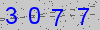# Formatting and Editing Math Equations

Mathematics. Maybe you dread it. Maybe you love it. Whatever your stance, math permeates everything. It even dictates your sleep. To start off the editing tip of the month, we wanted to bring forth a topic that will affect almost every author here at Accdon Letpub, no matter your discipline: the formatting and editing of equations.

Unsurprisingly, like many details in language editing, equations have a set of grammatical rules that need to be followed. The first, and arguably most important, rule deals with punctuation. Equations like to be a part of sentences; they don’t like being excluded. That being said, if there are multiple equations in a row, being presented as a list, actually treat them like a list: separate each equation from the other by a comma. Commas are also important to put after an equation if it is followed by a phrase beginning with “where.” (Here’s a good example of that.) Lastly, for punctuation, if the equation ends a sentence, put a period at the end of it.

Sometimes, journals rely on context and do not like putting in punctuation like this. If the target journal is not given, it is better to be safe and put in the punctuation. Make sure to add the punctuation to the manuscript properly (sometimes authors put in equations as JPEGs or in other difficult formats); make a note to our editor, so he/she can help you as needed.

Another important rule regarding equations deals with their introduction. Editors & authors around the globe are guilty of getting trigger-happy with colons when they introduce equations. Do not fall into that nasty trap, authors.

We digress: a colon is only necessary when introducing an equation if the sentence that is introducing said equation is complete. Below are two examples.

Correct: “We define the heat equation as follows: …”
Incorrect: “The heat equation is defined as: …”

Think of it this way, if you can’t put a period at the end of that introductory phrase and feel confident that it can stand alone as its own sentence, don’t tack a colon onto the end of it. (“The heat equation is defined as.” See how that doesn’t work?)

1. Penn State
2. UC Davis (This one is priceless: funny and extensive.)
3. A little teaser: if you are interested in learning about LaTeX, which is a typesetting language that allows you to write scientific symbols and formula inline (!), you can read about it here.

Happy Math-ing!

(Please retain the reference in reprint: http://www.letpub.com/index.php?page=author_education_formatting_and_editing_math_equations)

Security Code*